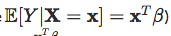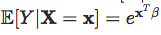{{announcement.body}}
{{announcement.title}}

DZone 's Guide to

### Are you a math nerd and lover of all things data science? Then this post if for you! Read on for the insights of a data expert.

· Big Data Zone ·
Free Resource

Comment (0)

Save
{{ articles.views | formatCount}} Views

Usually, when I give a course on GLMs, I try to insist on the fact that the link function is probably more important than the distribution. In order to illustrate, consider the following dataset, with 5 observations

``````x = c(1,2,3,4,5)
y = c(1,2,4,2,6)
base = data.frame(x,y)``````

Then consider several model, with various distributions, and either an identity link; and in that caseor a log link function, so that:``````regNId = glm(y~x,family=gaussian(link="identity"),data=base)

One can also consider some Tweedie distribution, to be even more general:

``````library(statmod)

Consider the prediction obtained in the first case, with the linear link function:

``````library(RColorBrewer)
darkcols = brewer.pal(8, "Dark2")
plot(x,y,pch=19)
abline(regNId,col=darkcols)
abline(regPId,col=darkcols)
abline(regGId,col=darkcols)
abline(regIGId,col=darkcols)
abline(regTwId,lty=2)``````The predictions are very very close, aren't they? In the case of the exponential prediction, we obtain:

``````plot(x,y,pch=19)
u=seq(.8,5.2,by=.01)
lines(u,predict(regNlog,newdata=data.frame(x=u),type="response"),col=darkcols)
lines(u,predict(regPlog,newdata=data.frame(x=u),type="response"),col=darkcols)
lines(u,predict(regGlog,newdata=data.frame(x=u),type="response"),col=darkcols)
lines(u,predict(regIGlog,newdata=data.frame(x=u),type="response"),col=darkcols)
lines(u,predict(regTwlog,newdata=data.frame(x=u),type="response"),lty=2)``````We can actually look closer. For instance, in the linear case, consider the slope obtained with a Tweedie model (that will include all the parametric familes mentioned here, actually).

``````pente=function(gamma) summary(glm(y~x,family=tweedie(var.power=gamma,link.power=1),data=base))\$coefficients[2,1:2]
Vgamma = seq(-.5,3.5,by=.05)
Vpente = Vectorize(pente)(Vgamma)
plot(Vgamma,Vpente[1,],type="l",lwd=3,ylim=c(.965,1.03),xlab="power",ylab="slope")``````The slope here is always very very close to one! Even more if we add a confidence interval:

``````plot(Vgamma,Vpente[1,])
lines(Vgamma,Vpente[1,]+1.96*Vpente[2,],lty=2)
lines(Vgamma,Vpente[1,]-1.96*Vpente[2,],lty=2)``````Heuristically, for the Gamma regression, or the Inverse Gaussian one, because the variance is a power of the prediction, if the prediction is small (here on the left), the variance should be small. So, on the left of the graph, the error should be small with a higher power for the variance function. And that's indeed what we observe here.

``````erreur=function(gamma) predict(glm(y~x,family=tweedie(var.power=gamma,link.power=1),data=base),newdata=data.frame(x=1),type="response")-y[x==1]
Verreur = Vectorize(erreur)(Vgamma)
plot(Vgamma,Verreur,type="l",lwd=3,ylim=c(-.1,.04),xlab="power",ylab="error")
abline(h=0,lty=2)``````Of course, we can do the same with the exponential models:

``````pente=function(gamma) summary(glm(y~x,family=tweedie(var.power=gamma,link.power=0),data=base))\$coefficients[2,1:2]
Vpente = Vectorize(pente)(Vgamma)
plot(Vgamma,Vpente[1,],type="l",lwd=3)``````Or, if we add the confidence bands, we obtain:

``````plot(Vgamma,Vpente[1,],ylim=c(0,.8),type="l",lwd=3,xlab="power",ylab="slope")
lines(Vgamma,Vpente[1,]+1.96*Vpente[2,],lty=2)
lines(Vgamma,Vpente[1,]-1.96*Vpente[2,],lty=2)``````So here also, the "slope" is rather similar... And if we look at the error we make on the left part of the graph, we obtain:

``````erreur=function(gamma) predict(glm(y~x,family=tweedie(var.power=gamma,link.power=0),data=base),newdata=data.frame(x=1),type="response")-y[x==1]
Verreur = Vectorize(erreur)(Vgamma)
plot(Vgamma,Verreur,type="l",lwd=3,ylim=c(.001,.32),xlab="power",ylab="error")``````So my point is that the distribution is usually not the most important point on GLMs, even if chapters of books on GLMs are distribution based. But, as mentioned in an another post, if you consider a nonlinear transformation, like we have with GAMs, the story is more complicated.

Topics:
big data, data modeling, r, tutorial

Comment (0)

Save
{{ articles.views | formatCount}} Views

Published at DZone with permission of Arthur Charpentier , DZone MVB. See the original article here.

Opinions expressed by DZone contributors are their own.